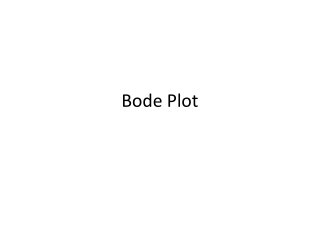Download PresentationBode Plot

# Bode Plot

Download Presentation## Bode Plot

- - - - - - - - - - - - - - - - - - - - - - - - - - - E N D - - - - - - - - - - - - - - - - - - - - - - - - - - -
##### Presentation Transcript

1. Bode Plot

2. Bode Plot for G(s)=s+a • Let s=jω, G(jω)=jω+a= • At low frequencies, when ω approach zero, G(jω)≈a The magnitude response in dB is 20log M=20log a, where , a constant from ω=0.01a to a. • At high frequencies, ω>>a, G(jω)≈ The magnitude response in dB is 20 log M=20 log a + 20 log =20 log ω, where , y=20x, straight line

3. We call the low frequency approximation and high frequency approximation with the term low frequency asymptote and high frequency asymptote respectively. • The frequency, a, is called the break frequency. • As for phase response, at low frequency , the phase=0o, at high frequency, phase=90o. • To draw the curve, start one decade (1/10) below the break frequency (0.1a) , draw a line of slope +45o/decade passing through 45o at the break frequency and continue to 90o at one decade above the break frequency (10a).

4. To normalize (s+a), we factor out a and form a[(s/a)+1]. • By defining a new frequency variable, s1=s/a, then the magnitude is divided by a to yield 0 dB at the break frequency. • The normalized and scaled function is (s1+1). • To obtain the original frequency response, the magnitude and frequency is multiplied by a.

5. Table 10.1Asymptotic and actual normalized and scaledfrequency response data for (s + a)

6. Figure 10.7Asymptotic and actual normalized and scaled magnitude response of (s+ a)

7. Figure 10.8Asymptotic and actual normalized and scaled phase response of (s + a)

8. Bode Plot for G(s)=1/(s+a) The function has a low frequency asymptote of 20log(1/a), when s approach zero. The Bode plot is constant until break frequency, a rad/s is reached. At high frequency asymptote, when s approach infinity,

9. Normalized and scaled Bode plots for a. G(s) = s; b. G(s) = 1/s;c. G(s) = (s + a); d. G(s) = 1/(s + a)

10. Draw the Bode Plots for the system shown below, G(s)=K(s+3)/[s(s+1)(s+2)] The break frequencies are at 1,2,3. The magnitude plot should begin a decade below the lowest break frequency and extend to a decade above the highest break frequency. Hence, we choose 0.1 rad to 100 rad for this plot. The effect of K is to move the magnitude curve up and down by the amount of 20log K. K has no effect on the phase curve. Let K=1 in this case.

11. Magnitude Plot:

12. Bode log-magnitude plot: a. components;b. composite

13. Phase Plot:

14. Bode Plot for The straight line is twice the slope of a first order term which is 40dB/decade.

15. Bode asymptotes for normalized and scaled G(s) =a. magnitude;b. phase

16. In second order polynomial, ωn is the break frequency. • For normalization, we divide the magnitude by ωn2, and scale the frequency , dividing by ωn . Thus, • G(s1) has a low frequency asymptote at 0dB and a break frequency of 1 rad/s. • For the phase plot, it is at 0o at low frequencies and 180o at high frequencies. The phase plot increase at a rate of 90o/decade from 0.1 to 10 and passes through 90o at 1. • The error between the actual response and the asymptotic approximation of the second order polynomial can be great depending on the value of ζ. • The actual magnitude and phase for are:

17. Bode Plot for

18. Draw the Bode log-magnitude and phase plots of G(s) for an unity feedback system.

19. Exercise: • Draw the Bode log-magnitude and phase plots for the system below: